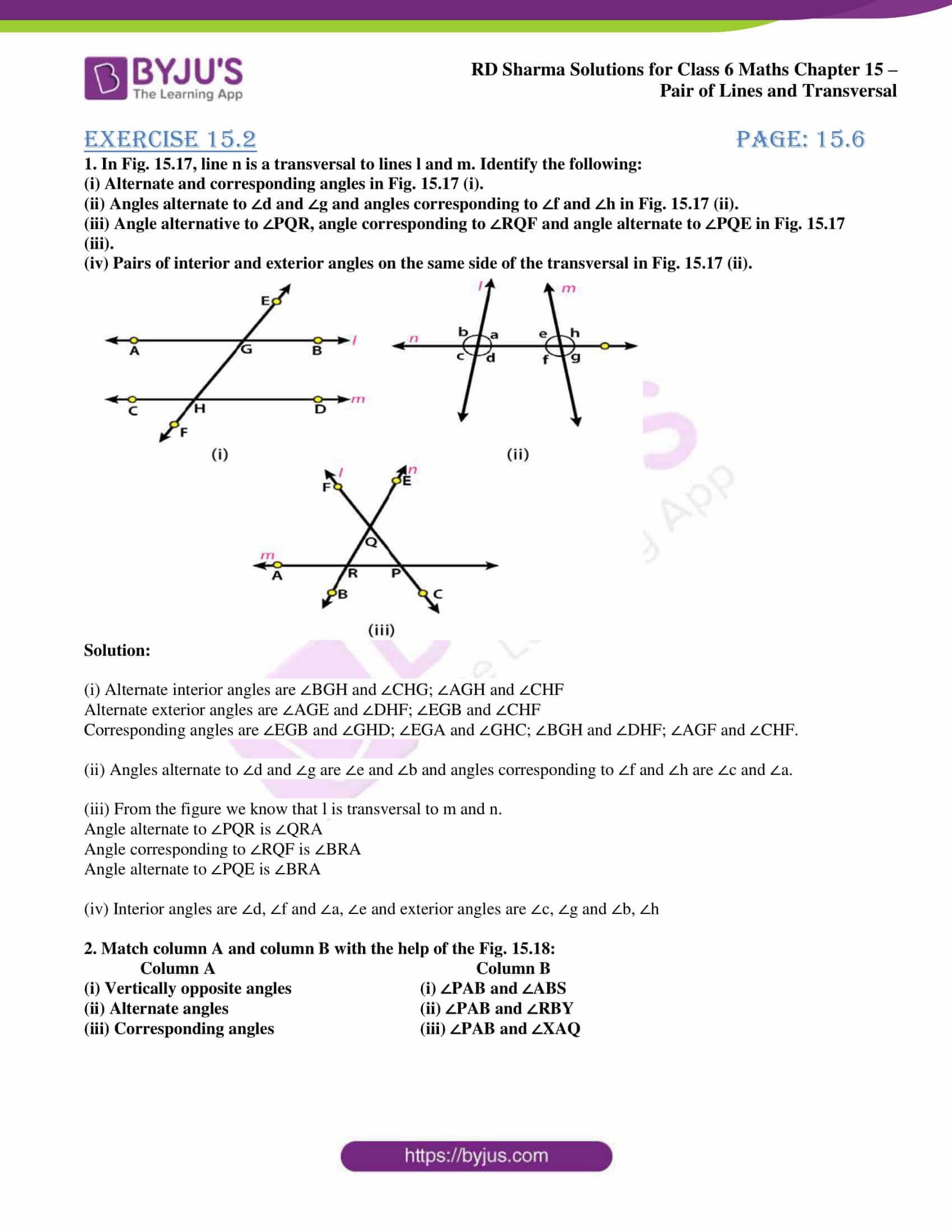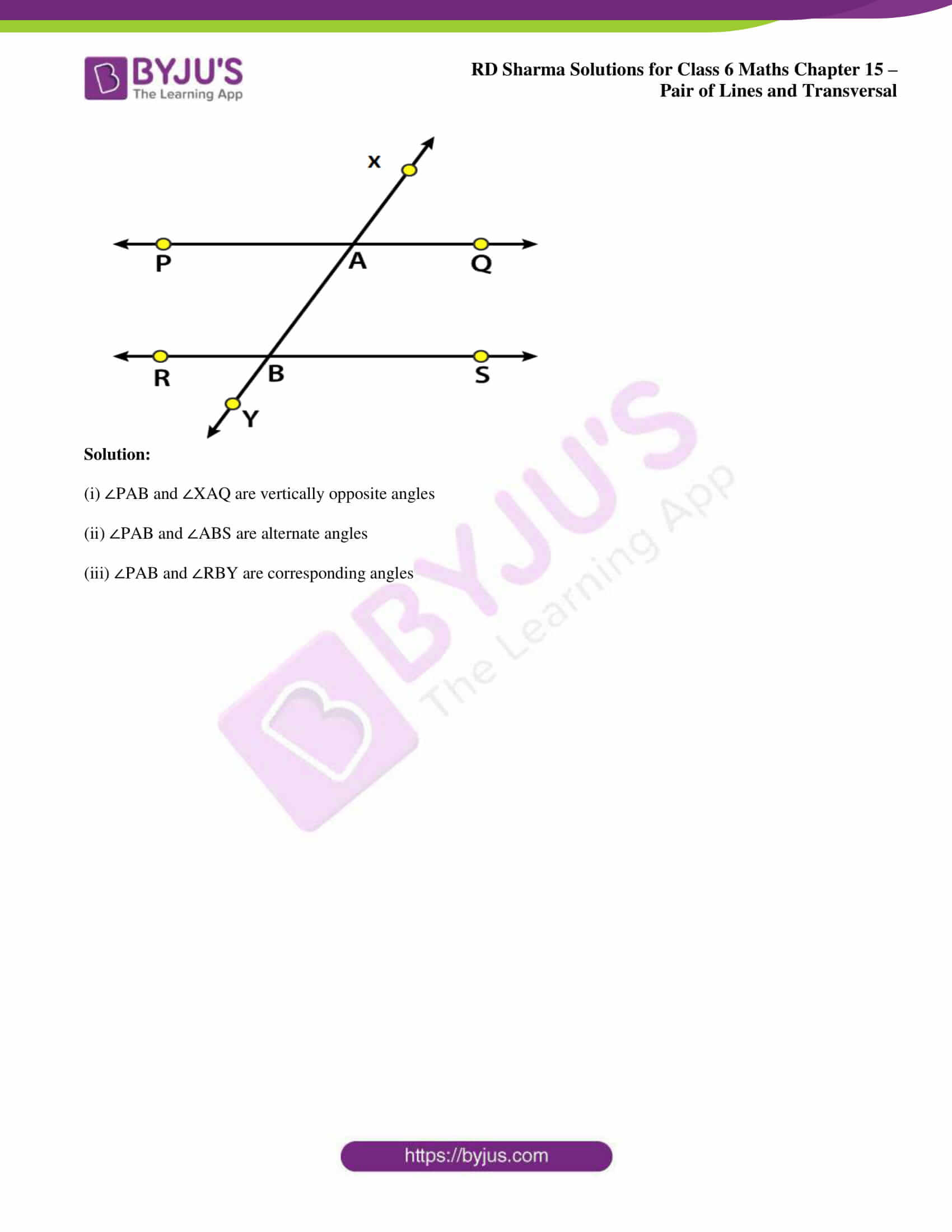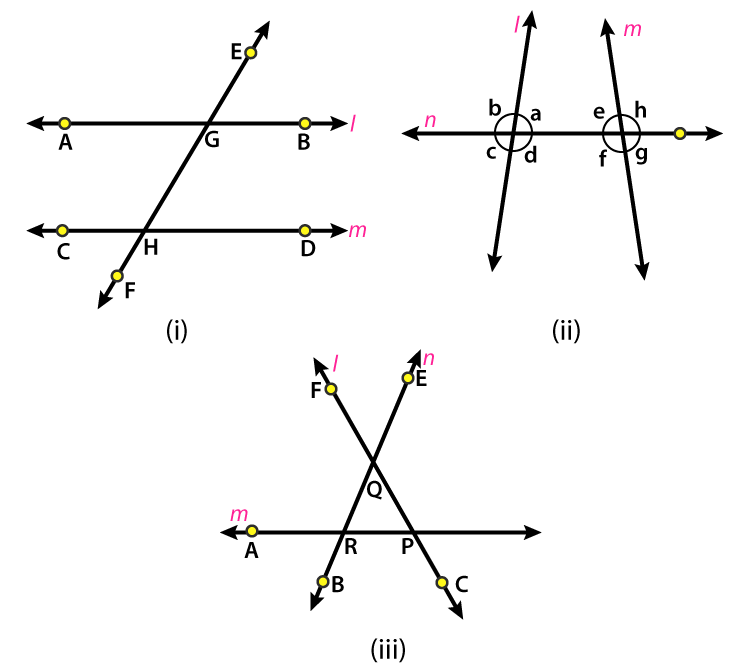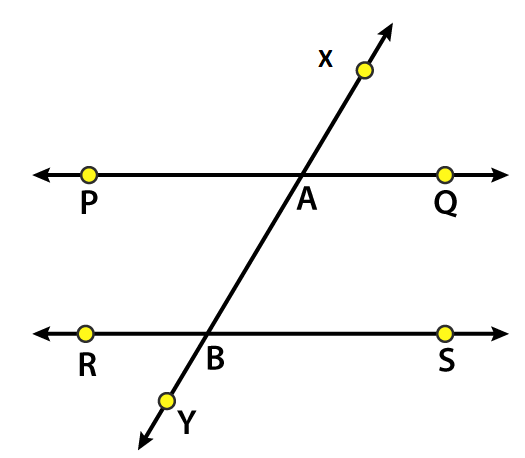# RD Sharma Solutions for Class 6 Maths Chapter 15: Pair of Lines and Transversal Exercise 15.2

The students can download the solutions and use them as a reference guide to get better conceptual knowledge. This exercise has questions based on transversal and angles by a transversal with two lines. Transversal is a line intersecting two or more lines in a plane at different points. The various angles formed by a transversal with two lines are interior angles, exterior angles, corresponding angles, and alternate interior and exterior angles. Links to RD Sharma Solutions Class 6 Maths Chapter 15 Pair of Lines and Transversal Exercise 15.2 PDF are available here.

## RD Sharma Solutions for Class 6 Maths Chapter 15: Pair of Lines and Transversal Exercise 15.2 Download the PDF### Exercise 15.2 page: 15.6

1. In Fig. 15.17, line n is a transversal to lines l and m. Identify the following:

(i) Alternate and corresponding angles in Fig. 15.17 (i).

(ii) Angles alternate to ∠d and ∠g and angles corresponding to ∠f and ∠h in Fig. 15.17 (ii).

(iii) Angle alternative to ∠PQR, the angle corresponding to ∠RQF and angle alternate to ∠PQE in Fig. 15.17 (iii).

(iv) Pairs of interior and exterior angles on the same side of the transversal in Fig. 15.17 (ii).Solution:

(i) Alternate interior angles are ∠BGH and ∠CHG; ∠AGH and ∠CHF

Alternate exterior angles are ∠AGE and ∠DHF; ∠EGB and ∠CHF

Corresponding angles are ∠EGB and ∠GHD; ∠EGA and ∠GHC; ∠BGH and ∠DHF; ∠AGF and ∠CHF.

(ii) Angles alternate to ∠d and ∠g are ∠e and ∠b, and angles corresponding to ∠f and ∠h are ∠c and ∠a.

(iii) From the figure, we know that l is transversal to m and n.

Angle alternate to ∠PQR is ∠QRA

Angle corresponding to ∠RQF is ∠BRA

Angle alternate to ∠PQE is ∠BRA

(iv) Interior angles are ∠d, ∠f and ∠a, ∠e and exterior angles are ∠c, ∠g and ∠b, ∠h

2. Match column A and column B with the help of Fig. 15.18:

Column A Column B

(i) Vertically opposite angles (i) ∠PAB and ∠ABS

(ii) Alternate angles (ii) ∠PAB and ∠RBY

(iii) Corresponding angles (iii) ∠PAB and ∠XAQSolution:

(i) ∠PAB and ∠XAQ are vertically opposite angles

(ii) ∠PAB and ∠ABS are alternate angles

(iii) ∠PAB and ∠RBY are corresponding angles